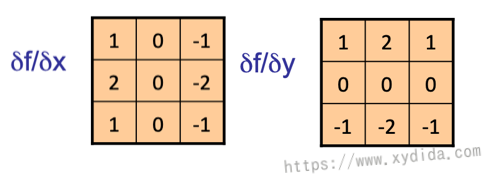# 数字图像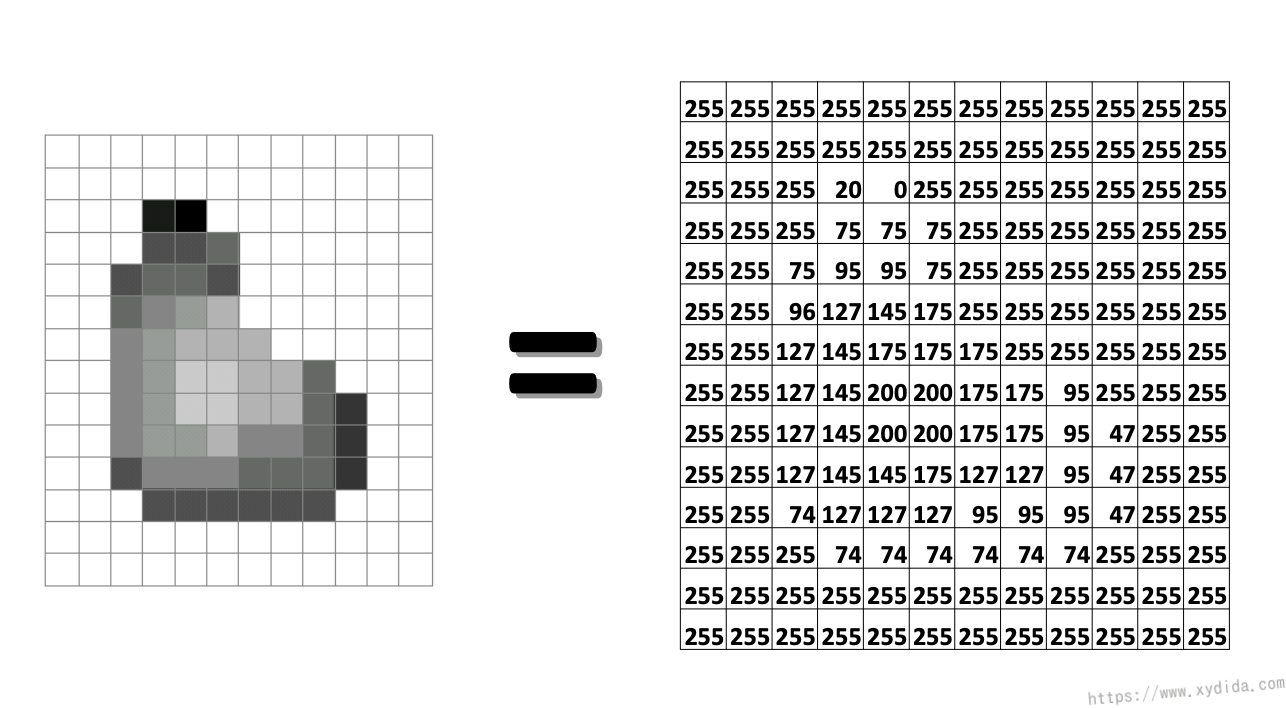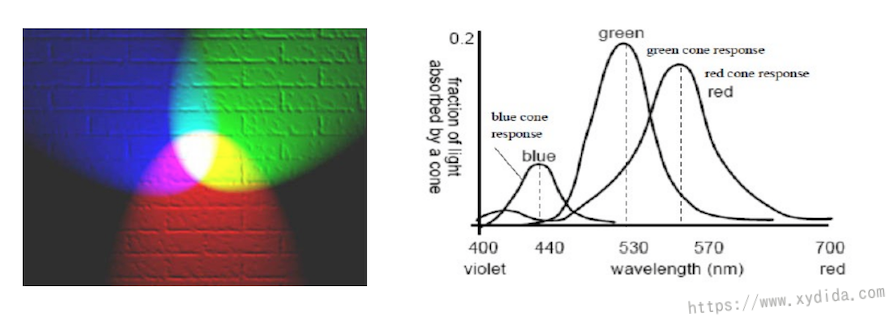$Y = 0.3(R) + 0.59(G) + 0.11(B)$

# 图像处理就是一种变换（transform）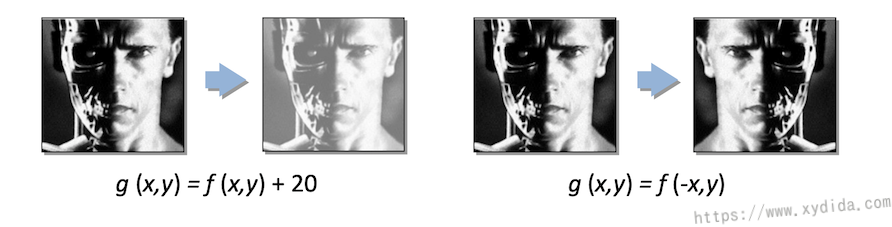• 实用，可以检测边缘，细节锐化，降噪等
• 配合卷积算子，效率高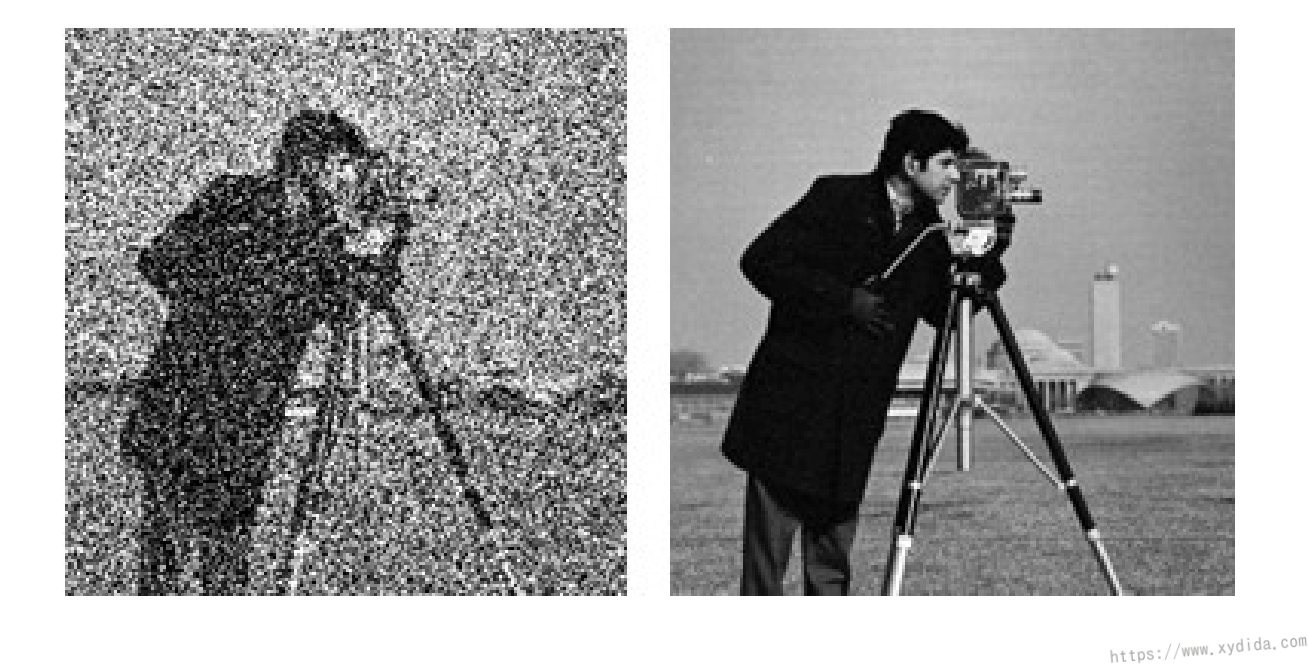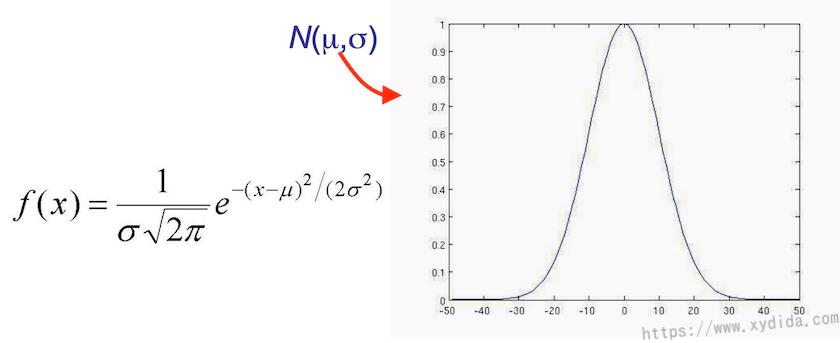# 降噪

## 多图像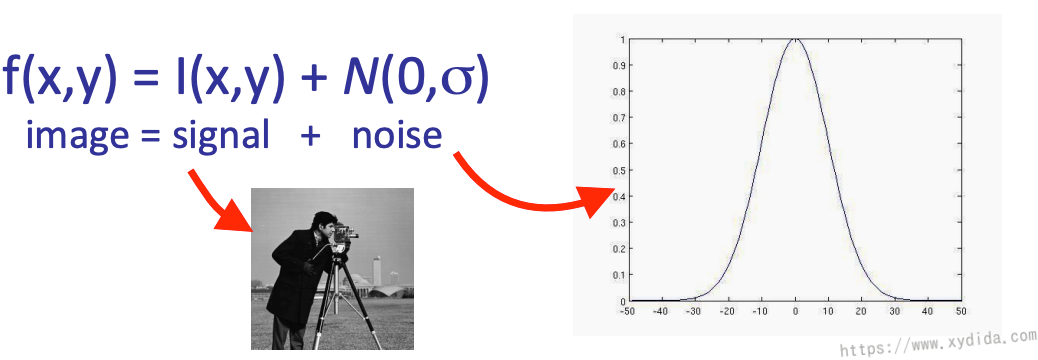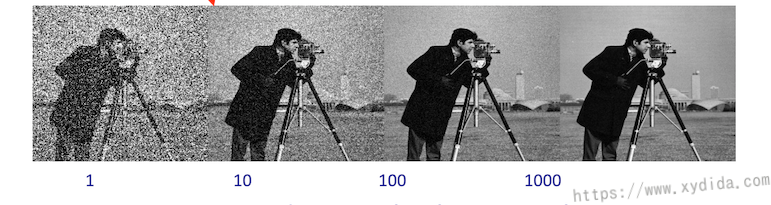## 单张图片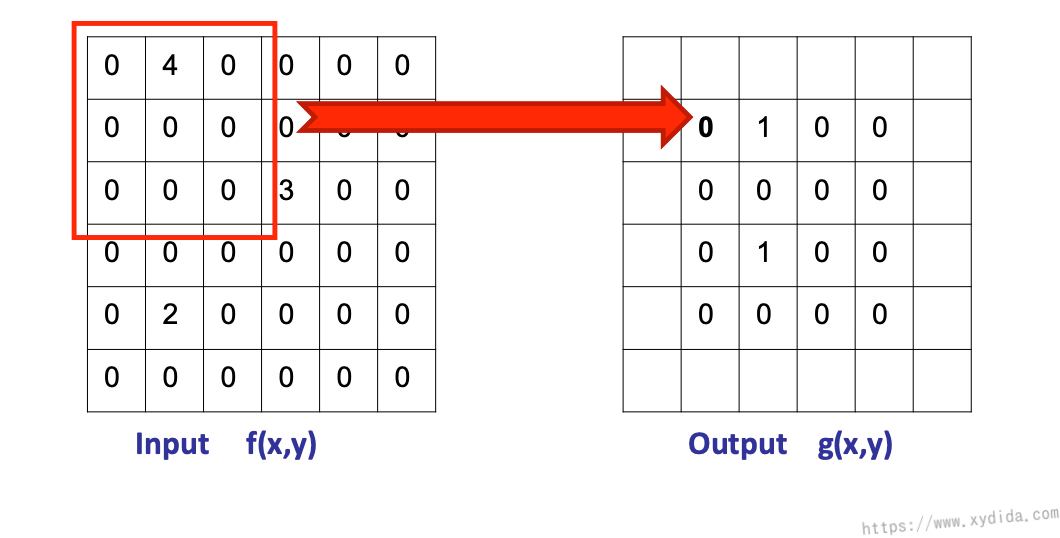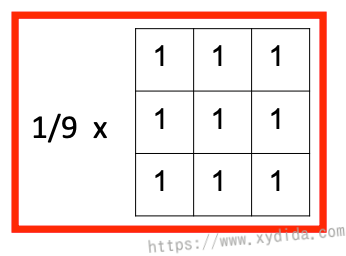$1/9 * (1*0 + 1*4 + 1*0 ...) = 4/9 = 0$

# 卷积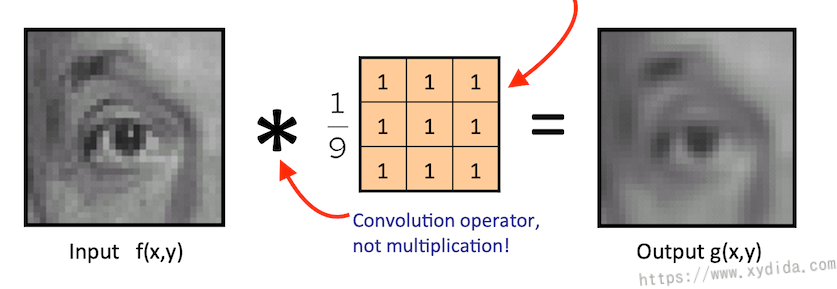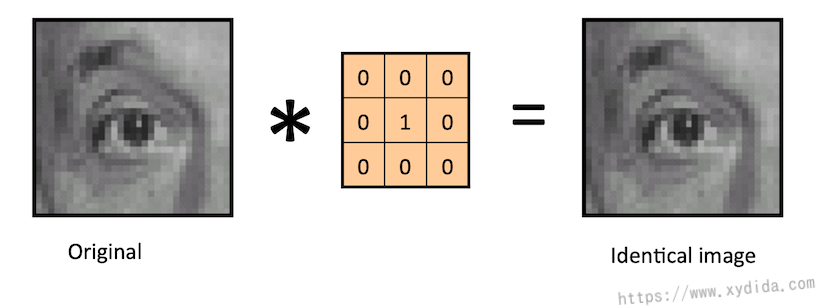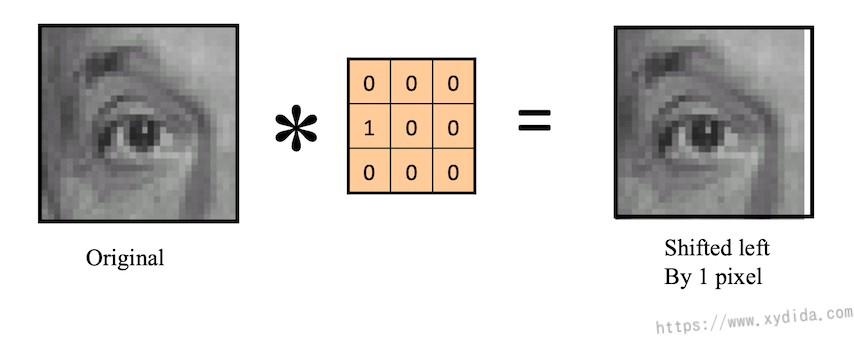## 一维数据卷积

f(x)为输入信号，h(x)为过滤器：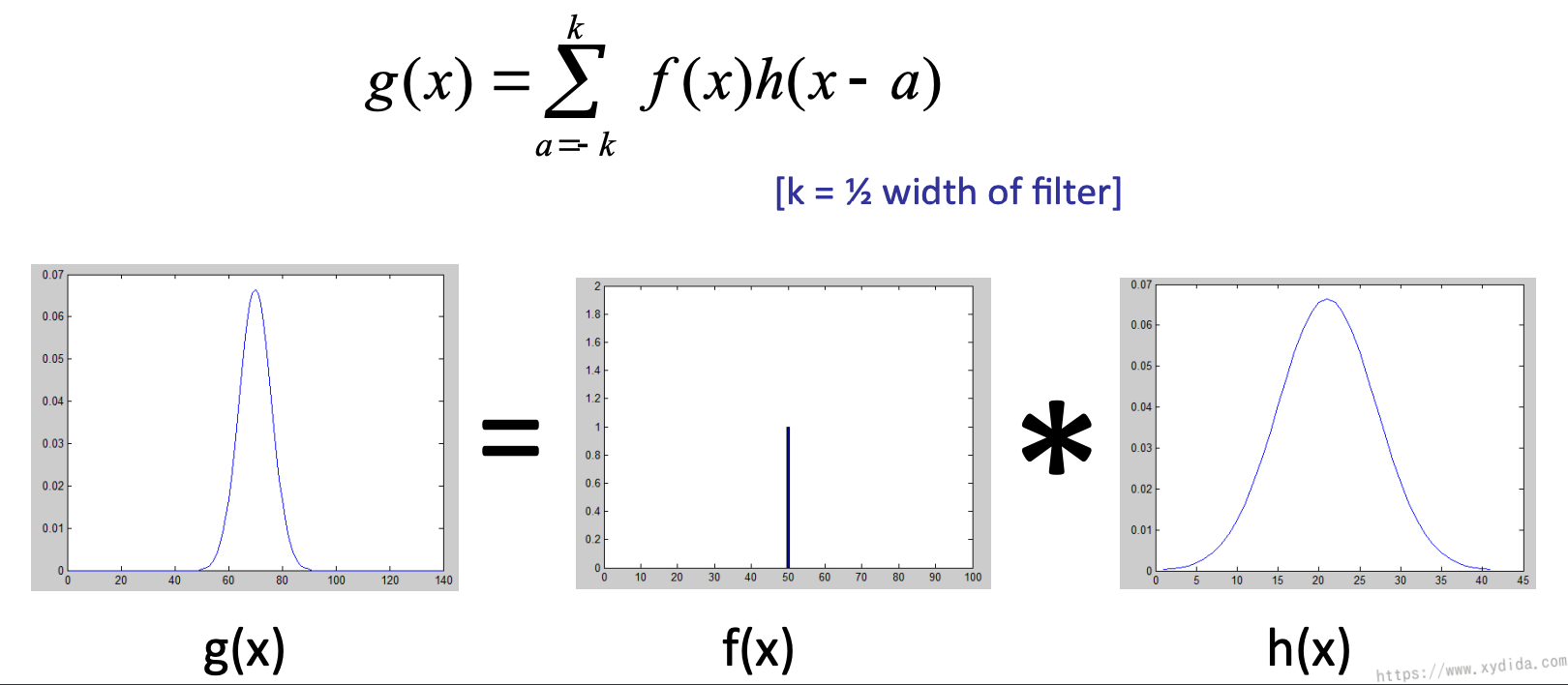## 二维数据卷积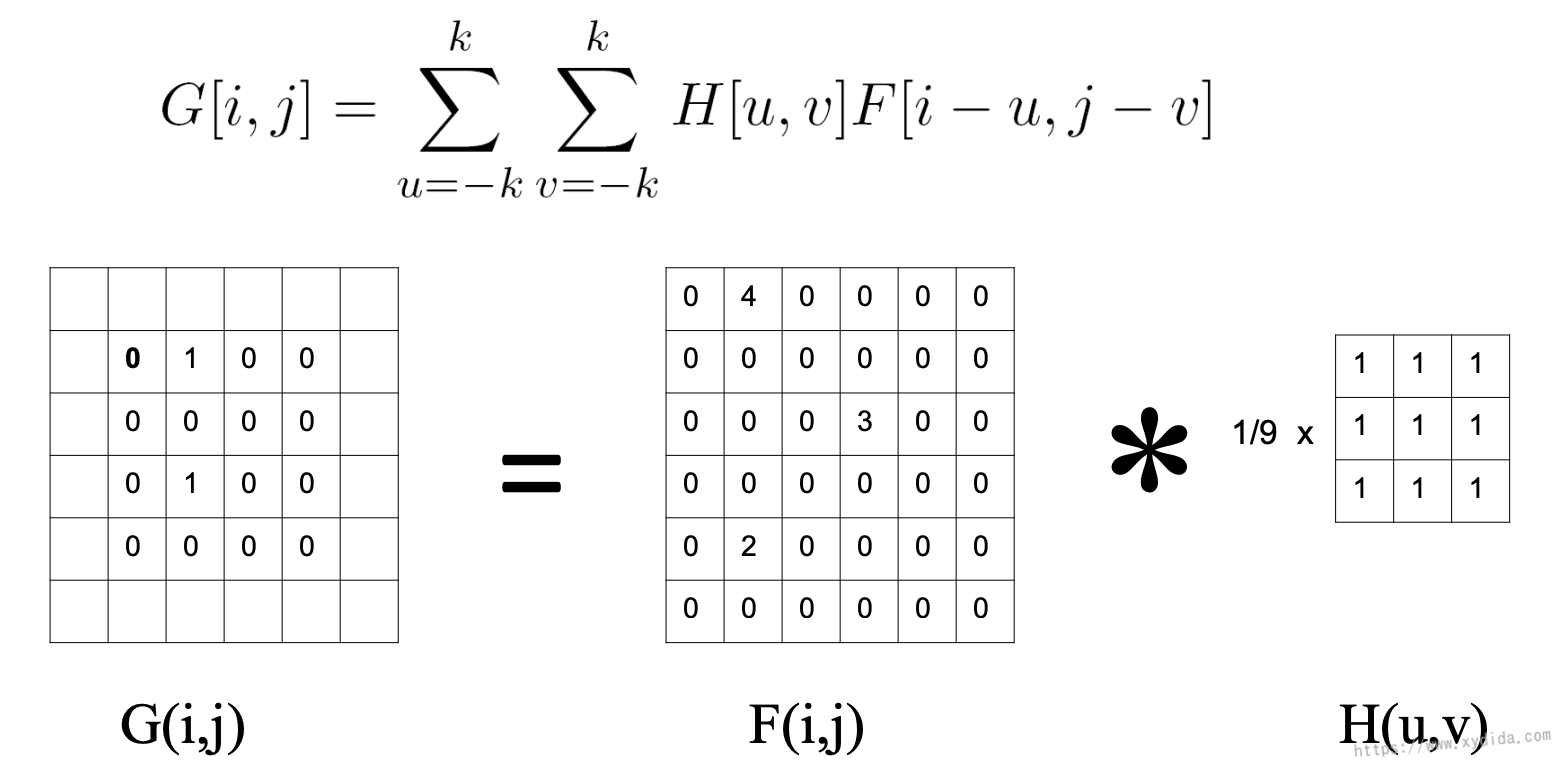# 图像模糊（blurring）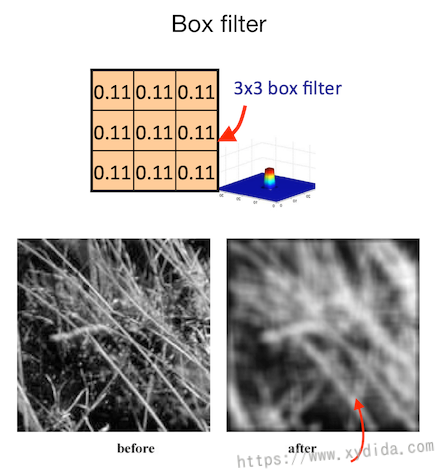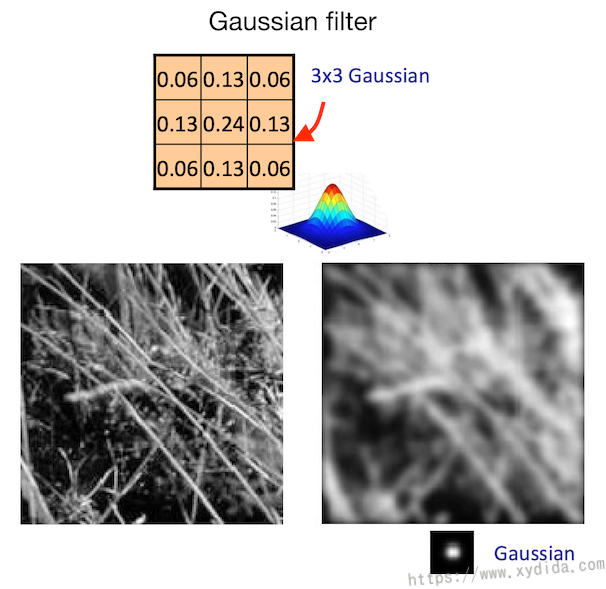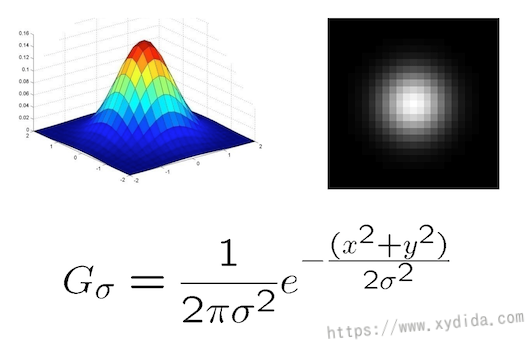# 边缘检测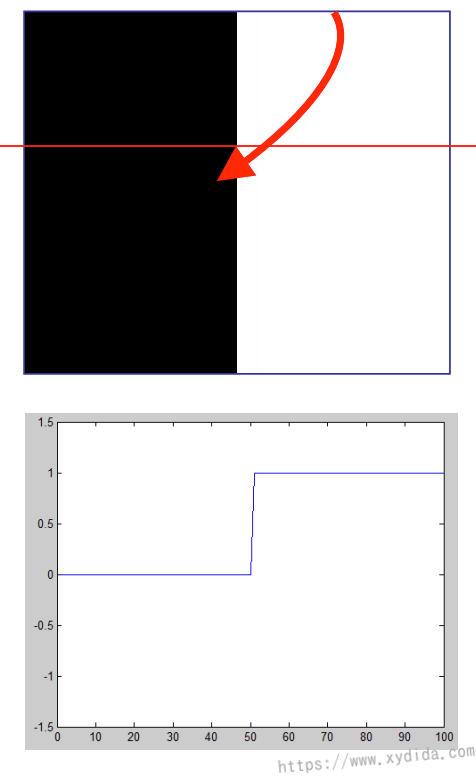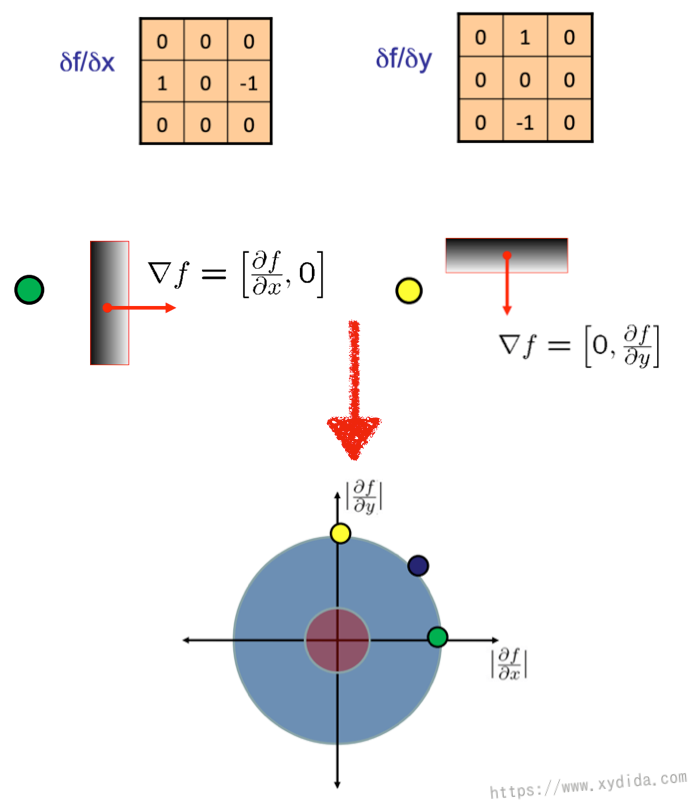$\lVert\nabla{f}\rVert = \sqrt{(\frac{\delta{f}}{\delta{x}})^{2} + (\frac{\delta{f}}{\delta{y}})^{2}} \tag{1}$

$\theta = tan^{-1}(\frac{\delta{f}}{\delta{y}} / \frac{\delta{f}}{\delta{x}}) \tag{2}$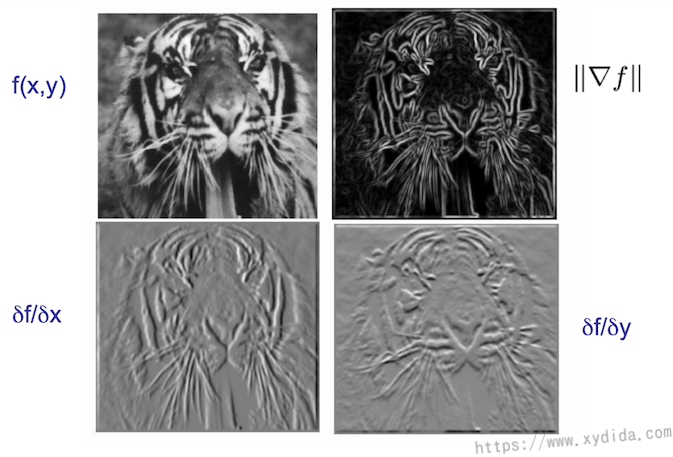## Sobel边缘算子

Sobel经常被用来检测边缘，同时它还有平滑图像的功能，算子如下：